# MAE/SimMIM for Pre-Training Like a Masked Language Model

In this post, I introduce a recently published method for self-supervised learning in a framework similar to masked language models. The two papers introduced in this article are MAE (He et al., (2021) and SimMIM (Xie et al., 2021). Each of them can be lightly summarized as follows.

The authors proposed MAE (Masked Autoencoders) which is a self-supervised learning method that masks an image and restores it. Even though it uses a ViT model that requires a large amount of data, it is possible to achieve 87.8% accuracy at ImageNet with ImageNet data alone. The performance is higher than existing self-supervised learning methods such as DINO and MoCo-v3,

This is astudy of pre-training by using transformers to perform the task of predicting masked areas of an image using regression. It has a very simple structure and can outperform existing self-supervised learning methods such as DINO.

1. Self supervised learning
2. Transformer and Vision Transformer
3. MAE and SimMIM
4. Conclusion

# Self-supervised learning method: SimCLR

First, let’s look at SimCLR as a representative of image self-supervised learning methods for computer vision tasks. SimCLR is one of the most popular models for self-supervised learning for computer vision in recent years.

Self-supervised learning is a learning method that obtains useful representations without the use of teacher labels. Usually, self-supervised learning is used as a pre-training method, aiming to obtain good performance in downstream tasks (tasks to be performed after pre-training), such as image classification and object detection.

Usually we evaluate the performance of self-supervised learning by fine-tuning the trained model or performing classification using linear regression of the obtained representation on downstream tasks such as image classification.

SimCLR and Contrastive Learning

This section briefly introduces SimCLR, one of the most popular methods for self-supervised learning in computer vision tasks. The SimCLR uses a framework called contrastive learning to learn good representations of images without labels.

In contrastive learning, first, create paired image data with applying different data augmentations for each image. Next, update the weights of the network so that the representations of the paired image data are closer together and that of the unpaired data are further apart.

For example, the image below illustrates the concept of contrastive learning using a chair and a dog. The chair and the dog are each subjected to two different data augmentations, and the paired images are learned to move closer in the representation space if the source is the same image, and to move away if the source is different.

In SimCLR, a very good representations of the images are obtained using contrastive learning. The following figure shows the result of a linear regression using the obtained representations of the images. Even with a very simple linear regression model, the accuracy is comparable to that of supervised (ResNet50) results, which means that the image information is compressed very well.

The strategy of “creating paired image data with applying different data augmentation and comparing the output”, as contrastive learning, is widely used in self-supervised learning for computer vision tasks.

Similar examples using contrastive learning include MoCo v2 (Chen et al., 2020), and those not using contrastive learning include BYOL (Grill et al, 2020) and DINO (Caron et al., 2021).

DINO is a self-supervised learning method that uses vision transformers and distillation, as described below. Unlike SimCLR, DINO does not use contrastive learning, but it shares the same strategy as SimCLR, which is to create paired image data with applying different data augmentation and compare the output.

Such a strategy of “creating paired image data with applying different data augmentation and comparing the output” is widely used in image based self-supervised learning methods.

# Vision Transformer

First of all, I would like to explain ViT (Vision Transformer), which is the subject of comparison in this paper, and the transformer it is based on. So let’s start with the transformer.

The Transformer is a model proposed in the paper “Attention Is All You Need” (Vaswani et al., 2017). It is a model that uses a mechanism called self-attention, which is neither a CNN nor an LSTM, and builds Transformer model to outperform existing methods significantly. The results are much better than the existing methods.

Note that the part labeled Multi-Head Attention in the figure below is the core part of the Transformer, but it also uses skip-connection like ResNet.

The attention mechanism used in the Transformer uses three variables: Q (Query), K(Key), and V (Value). Simply put, it calculates the attention weight of a Query token (token : something like a word) and a Key token and multiplies the Value associated with each Key. In short, it calculates the association (attention weight) between the Query token and the Key token and multiplies the Value associated with each Key.

Defining the Q, K, V calculation as a single head, the multi-head attention mechanism is defined as follows. The (single-head) attention mechanism in the above figure uses Q and K as they are. Still, in the multi-head attention mechanism, each head has its projection matrix W_i^Q, W_i^K, and W_i^V, and they calculate the attention weights using the feature values projected using these matrices.

If the Q, K, V used in this attention mechanism are calculated from the same input, it is specifically called Self-Attention. On the other hand, the upper part of Transformer’s decoder is not a “self-” attention mechanism since it calculates attention with Q from the encoder and K and V from the decoder.

The image of the actual application is shown in the figure below. The figure shows a visualization of the attention weights calculated for each Key token using the word “making” as a query. The transformer uses a multi-headed self-attention mechanism to propagate to later layers, and each head learns different dependencies. The Key words in the figure below are colored to represent the attentional weight of each head.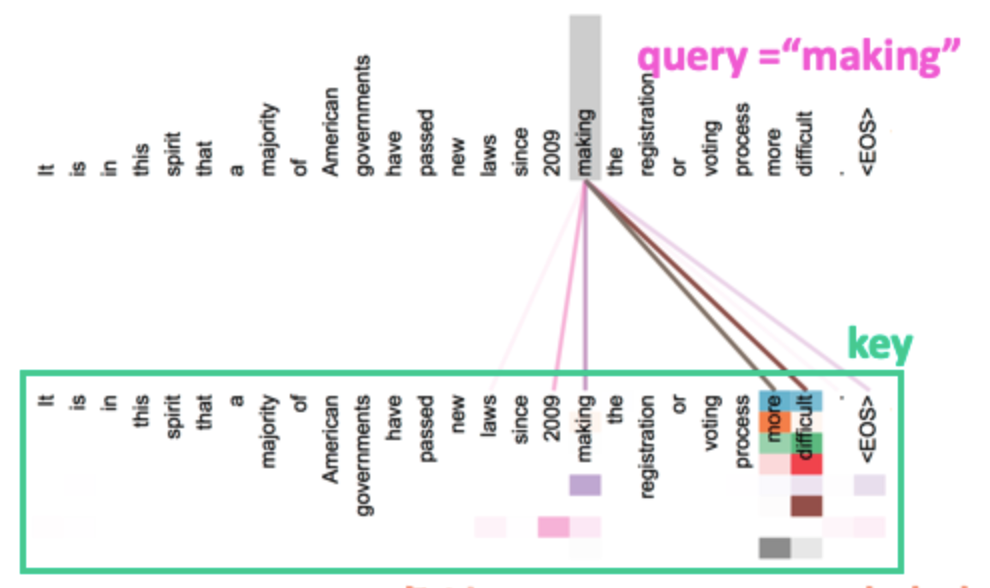Attention Weights visualization. The image quated from Vaswani et al., 2017 and I have annotated it.

Vision Transformer (ViT) is a model that applies the Transformer to the image classification task and was proposed in October 2020 (Dosovitskiy et al. 2020). The model architecture is almost the same as the original Transformer, but with a twist to allow images to be treated as input, just like natural language processing.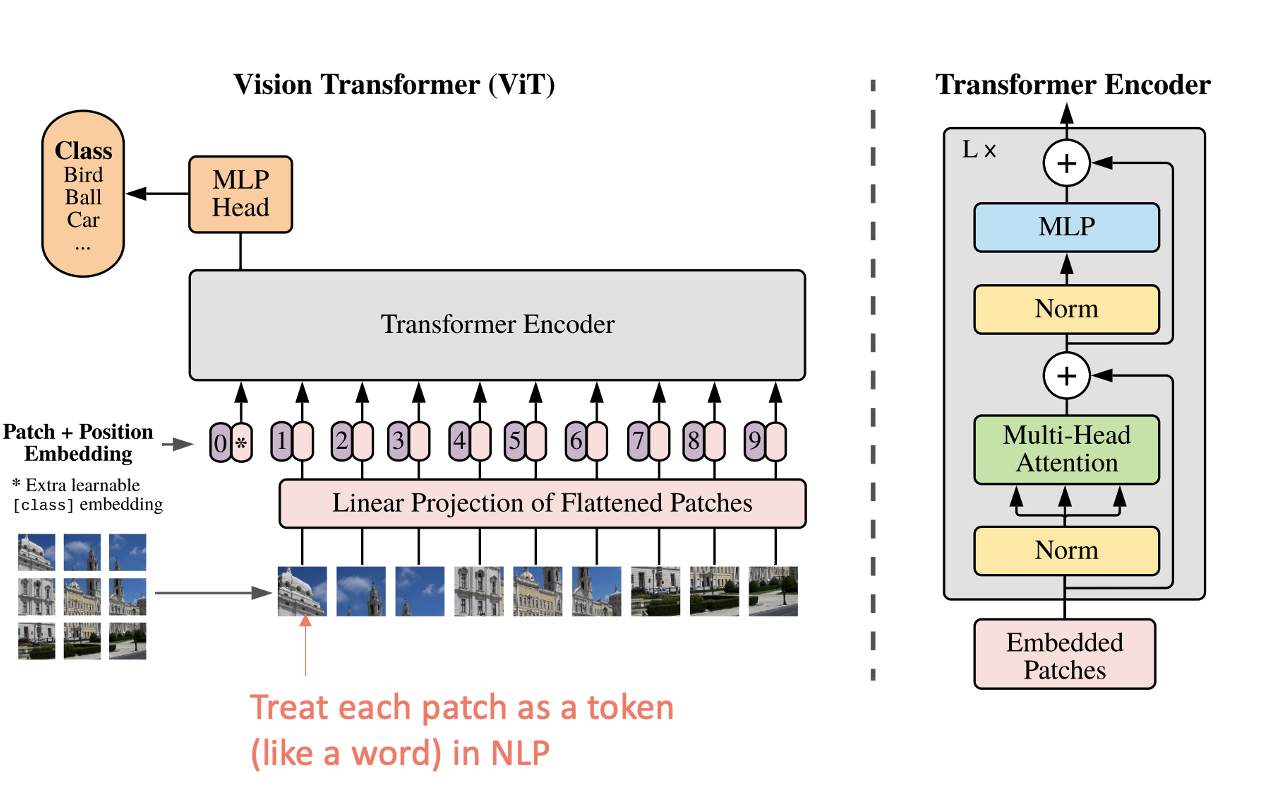Vision Transformer architecture. The image is quoted from Dosovitskiy et al. 2020 and I have annotated it.

First, ViT divides the image into N “patches” of such as 16x16. Since the patches themselves are 3D data (height x width x number of channels), they cannot be handled directly by a transformer that deals with language (2D), so it flattens them and makes a linear projection to convert them into 2D data. So each patch can be treated as a token, which can be input to the Transformer.

In addition, ViT uses the strategy of pre-training first and then fine-tuning. ViT is pre-trained with JFT-300M, a dataset containing 300 million images, and then fine-tuned on downstream tasks such as ImageNet. ViT is the first pure transformer model to achieve SotA performance on ImageNet, and this has led to a massive surge in research on transformers as applied to computer vision tasks.

However, training ViT requires a large amount of data. Transformers are less accurate with less data, but become more accurate with more data, and outperform CNNs when pre-trained on the JFT-300M. For more details, please refer to the original paper.

# Self-supervised learning methods such as masked language models for images

Finally, I will introduce two methods, MAE and SimMIM, which can be called the “computer vision version of masked language models”.

As mentioned above, the mainstream of self-supervised learning for computer vision tasks has been self-supervised learning using the strategy of “creating paired image data with applying different data augmentation and comparing the output”. These two methods, however, use a strategy of “masking a part of the image and predicting that” for self-supervised learning like a masked language model.

To begin with, MAE (Masked Autoencoders) is the model, which was published on November 11, 2021. MAE divides the image into patches and performs the task of predicting the masked parts of the image as pre-training. Characteristically, the decoder is fed with the input including the masked parts to restore the original image, but the encoder is not fed with the masked parts.

Let’s take a look at the model structure. First, for the encoder part, the it uses transformers. The masked part is not input to the encoder, so the advantage seems to be that we can use a huge model while saving memory. In this paper, the masking rate is about 75%, and considering that the memory of the self-attentive system is proportional to the fourth power of the image size, it uses only 1/16 (1/4²) of the memory compared to the case where the entire image is included.

The decoder also uses transformers, but it is much lighter than the encoder, and each token requires less than 10% of the computation of the encoder. Note that the decoder is only used to pre-train the mask partial reconstruction.

First, let’s look at the image reconstruction task (pre-training task). In this experiment, the results are the image reconstruction of the ImageNet validation set. We can see that the image is successfully reconstructed even though 80% of the image is masked.

Next, let’s take a look at the effect of mask ratio. The figure below shows an experiment of mask ratio and accuracy. We can see that the higher the mask ratio is, the better the results are in the downstream task, the image classification task.

Next, let’s take a look at the results of the downstream tasks. The first one is image classification. It gives excellent results compared to the self-supervised learning method using ViT.

Finally, there is object detection and semantic segmentation. This one also outperforms existing self-supervised learning methods and supervised learning.

# SimMIM

Next, I introduce SimMIM, which was announced on November 18, 2021. Like MAE, SimMIM masks the image. However, unlike MAE, SimMIM also includes the masked image in the encoder, and uses it as a direct prediction mechanism. It is very similar to MAE, but MAE is not listed as a reference, probably because it was published very close to MAE.

The model architecture is not complicated, and the transformer-based models ViT and Swin (Liu et al, 2021) can be used. The “One-layer Prediction Head”, which is a decoder in MAE, uses a simple linear model.

Although transformer-based decoders like MAE (e.g. Swin-T) have been tried, the simplest linear model outperformed in terms of both accuracy and computational cost.

First, here are the results of the image classification. In the fine-tuning results, SimMIM outperforms supervised learning.

Next, let’s look at masking methods. Figure 3 below shows an investigation of how masking methods affect representation learning. The authors use a metric called AvgDist to examine the impact of mask strategies. AvgDist is the average distance between the masked pixels and the visible pixels.

Figure 3(b) shows that AvgDist is not good when it is too high or too low. The authors speculate that this is due to the fact that a high AvgDist makes traiing too difficult, while a low AvgDist makes it too easy.

BERT (Devlin et al., 2018]) is a NLP model that takes a pre-training method that masks the data and guesses at it (masked language model). MAE and SimMIM also tokenize images (because they are based on ViT), so like BERT, the task is that masking and predicting token.

However, BERT uses a low mask ratio of 15%, while MAE and SimMIM use a high mask ratio of 50–80%. It is not directly discussed in the papers why high mask ratio is effective unlike BERT, but it may be because unlike documents, images have a two-dimensional structure, so it is possible to learn even with high mask ratio since the masked area can be inferred from both horizontal and vertical information.

# Conclusion

In this post, I introduced MAE and SimMIM, which employ a strategy of “masking a part of the image and predicting that” like a masked language model, as opposed to the conventional strategy of “creating paired image data with applying different data augmentation and comparing the output”.

Since these methods are easily used with ViT-based models, which have been gaining momentum in recent years, masked language model-like pre-training methods may become more widespread in the future.

--

--

--

Love podcasts or audiobooks? Learn on the go with our new app.

## Can Driver Alertness Be Predicted?## My convolutional neural network project — how did I make it “Cooler”?## Deploy and Serve AI Models (Part 2 — TensorFlow Serving)## Developing a simple CNN for MNIST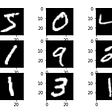## Machine Learning-An overview.## Logistic Regression In Machine Learning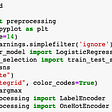## Low Resolution MRI for Deep Learning Models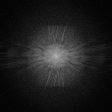## Autonomous Mars Rover using Raspberry Pi, Arduino and Pi Camera## Paper Summary: Masked Autoencoders Are Scalable Vision Learners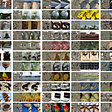## Review — Multi-task Self-Supervised Visual Learning## Sharpness-Aware Minimization for Efficiently Improving Generalization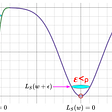## Warp speed model training in PADL with PyTorch-Lightning